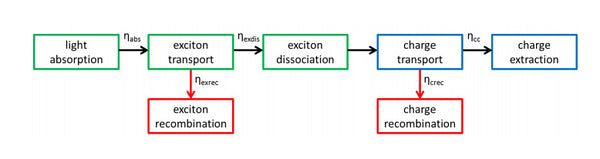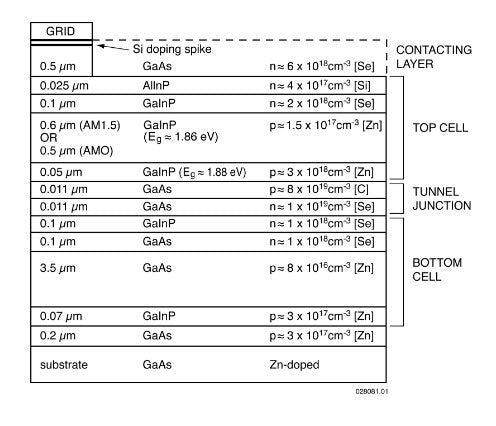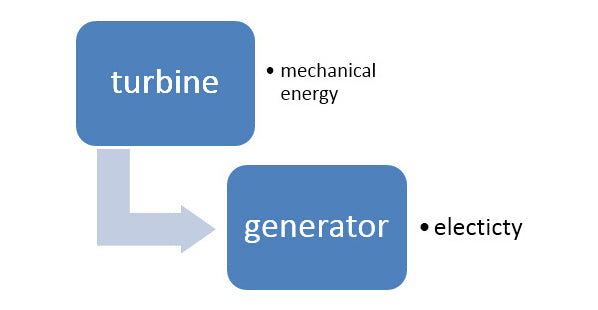# Why is the Solar Energy Conversion Efficiency so Low and Difficult to Improve?

Most PV panels now have an efficiency of 11-19%. Relatively low.

Solar energy conversion efficiency is divided into photoelectric conversion efficiency and photothermal conversion efficiency.

1. Photoelectric conversion efficiency
1. First of all, we must understand light. On the macro level, the sunlight we see is colorful, that is, light of different wavelengths. At the micro level, different rays of light are different frequencies of light. The different frequencies of light are because they carry different photons. The source of energy that can generate electricity is photons.

Photons energy: E=hc/λ, h is the Planck constant, c is the speed of light, λ is the wavelength

2. Bandgap, originated from energy band theory, is a term for studying the movement of electrons in solids. The energy band gap is the minimum energy required to release electrons from the bond. If the energy carried by the photon is less than the energy necessary for the band gap, electrons cannot be released. Taking crystalline silicon as an example, the bandgap of Si is about 1.12ev, which is about 1.1 wavelengths converted into photons. Then the spectrum of sunlight formed on the surface of the earth is 2u-400nm. From the formula just now, we can see that the shorter the wavelength, the greater the energy.

In general, the photoelectric process is that photons split electrons from atomic bonds to produce charge carriers. PV panels are semiconductors. Semiconductor charge carriers contain negative electrons (negatively charged electrons) and positive holes (positively charged holes).The reasons for low efficiency are divided into three steps:
1. When the light hits the PV panel, a part of it will be reflected and not incident on the PV;

2. Some photons have insufficient energy (the wavelength is too large) to reach the bandgap. It is conservatively estimated that 25% of incident natural light is not absorbed because its energy value is lower than the band gap of crystalline silicon, and no photoelectric conversion occurs at all.

3. Some photons have too much energy, causing some to enter the PV to generate heat. The reason for the heat generation is that some of the photons carry too much energy to directly transfer heat without generating electricity. It is conservatively estimated that it accounts for 30% and the last remaining part is completed before photoelectric conversion.
Therefore, the main reason for the low efficiency of PV panels is that they cannot actively reflect all spectra.

other reasons:
1. The materials used in the PV panel are different, and the natural resistance of the material itself to the electron flow is also different. This natural resistance will also reduce the PV efficiency.

2. There will be losses in the board and electrical contacts.

3. The best efficiency of solar cells is at low temperature, and the efficiency will decrease as the temperature rises. The dramatic thing is that the PV panels have to be exposed to the sun all the time, so the efficiency is greatly reduced

4. The PV panel cannot face the light all day, and its angle is lost.

Now the latest efficiency can be increased to 44.7%. Use multi-layer coating. But it is too expensive and is generally only used in aerospace.2. Photothermal conversion efficiency. I have answered this part before and simplified it.

Also known as CSP, focused power generation. Leave storage aside.

Mirror heat collection-(oil or water)-steam-steam turbine-power generation

The efficiency here involves heat transfer efficiency, steam conversion efficiency, Carnot cycle efficiency and Rankine cycle efficiency.

The main loss:

1. Loss of steam turbineThe 40% efficiency of the steam turbine reduces the power generation efficiency.

2. The heat transfer efficiency will lose 40%

3. Heat-steam efficiency will be lost> 10%

Therefore, the overall power generation efficiency of CSP is about 30%.